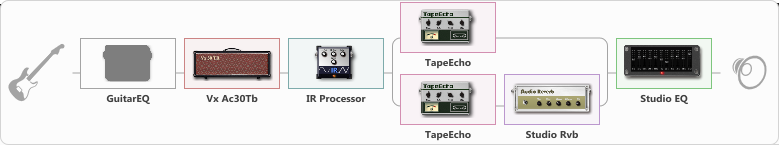# Amapola - The Spotnicks (Bo Winberg)

Discussion in 'ToneLib-GFX presets' started by eddy.grand, Jul 5, 2020.

1. Amapola - The Spotnicks (Bo Winberg)
Preset name: Amapola - The Spotnicks

Stratocaster, Bridge-PU.
Hope, You like it.

Effects chain:Effect: "GuitarEQ" (Dynamics / Filter), active - "no"
{

"160 Hz" = 0
"400 Hz" = 0
"800 Hz" = 0
"1.6 kHz" = 0
"3.2 kHz" = 0
"6.4 kHz" = 0
"12 kHz" = 0
"Level (dB)" = 0
}

Effect: "Vx Ac30Tb" (Amp simulators), active - "yes"
{

"Gain" = 20
"Bass" = 100
"Middle" = 86
"Treble" = 60
"Presence" = 100
"Master" = 80
"Level (dB)" = 8
}

Effect: "IR Processor" (Cabinets), active - "yes"
{

"IR" = 57 edge grill
"Low Cut (Hz)" = 28
"Hi Cut (kHz)" = 7.5
"Mix" = 100
"Level (dB)" = 0
}

Effect: "Splitter" (Dynamics / Filter), active - "yes"
{

"A-Bypass" = Off
"A-Pan" = 0
"A-Level" = 55
"B-Bypass" = Off
"B-Pan" = 0
"B-Level" = 55
'A' branch:
{

Effect: "TapeEcho" (Delay), active - "yes"
{

"Time" = 108
"Feedback" = 27
"Tone" = 48
"LoDamp" = 51
"Mix" = 66
}
}
'B' branch:
{

Effect: "TapeEcho" (Delay), active - "yes"
{

"Time" = 216
"Feedback" = 0
"Tone" = 49
"LoDamp" = 49
"Mix" = 72
}
Effect: "Studio Rvb" (Reverb), active - "yes"
{

"Time" = 9.0
"PreDelay" = 28
"LoDamp" = 0
"HiDamp" = 80
"Mix" = 85
}
}
}

Effect: "Studio EQ" (Dynamics / Filter), active - "yes"
{

"31 Hz" = 9
"62 Hz" = 14
"125 Hz" = 3
"250 Hz" = 0
"500 Hz" = 5
"1 kHz" = 0
"2 kHz" = 0
"4 kHz" = 0
"8 kHz" = 0
"16 kHz" = 0
"Level (dB)" = 0
}

Note: You will need to download and install the ToneLib-GFX software to use the preset.

#### Attached Files:

• ###### Amapola_-_The_Spotnicks_Bo_Winberg.tlgfx
File size:
4.2 KB
Views:
9,599
Last edited: Apr 15, 2021
2. Many thanks Mic Vanessche!

3. Thank you Sandy Marko Knauer!

4. Thanks, oblnsky!

5. Many thanks Krzysztofelek!

6. Thanks ktaxi!

7. Thanks Krzysztofelek!

8. Thank you IrvingPipo!

9. Thanks Matheus Scarpatti!

10. Thank you SEMENOV!

11. Thanks capoman!